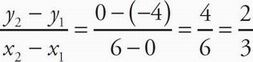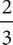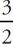# SAT Math Multiple Choice Question 141: Answer and Explanation

### Test Information

Question: 141

6.The graph, y = f(x), shown above models the performance of a certain crop, where x is the nutrients subtracted or added to the soil and y is the gain or loss of pieces of fruit added to the total harvest. A more powerful fertilizer that is used causes the graph y = f(x) to be reflected over the line y = x. Which of the following best describes the behavior of the crop with the new fertilizer?

• A. For every three nutrients added to the soil, the crop loses two additional fruits for the total harvest.
• B. For every two nutrients added to the soil, the crop loses two additional fruits for the total harvest.
• C. For every three nutrients added to the soil, the crop adds two additional fruits to the total harvest.
• D. For every two nutrients added to the soil, the crop adds three additional fruits to the total harvest.

D Start by finding the slope of the line provided on the graph using the points (0,-4) and (6,0) and the point-slope formula:. When this line is reflected across the line y = x, the x and y values switch, so the new slope would be the reciprocal of the original slope. Since our original slope was, our new slope will be. The numerator here reflects the gain or loss of pieces of fruit in the harvest, and the denominator reflects the nutrients subtracted or added. This means that for every two nutrients added, there will be a harvest gain of three pieces of fruit, which is (D).